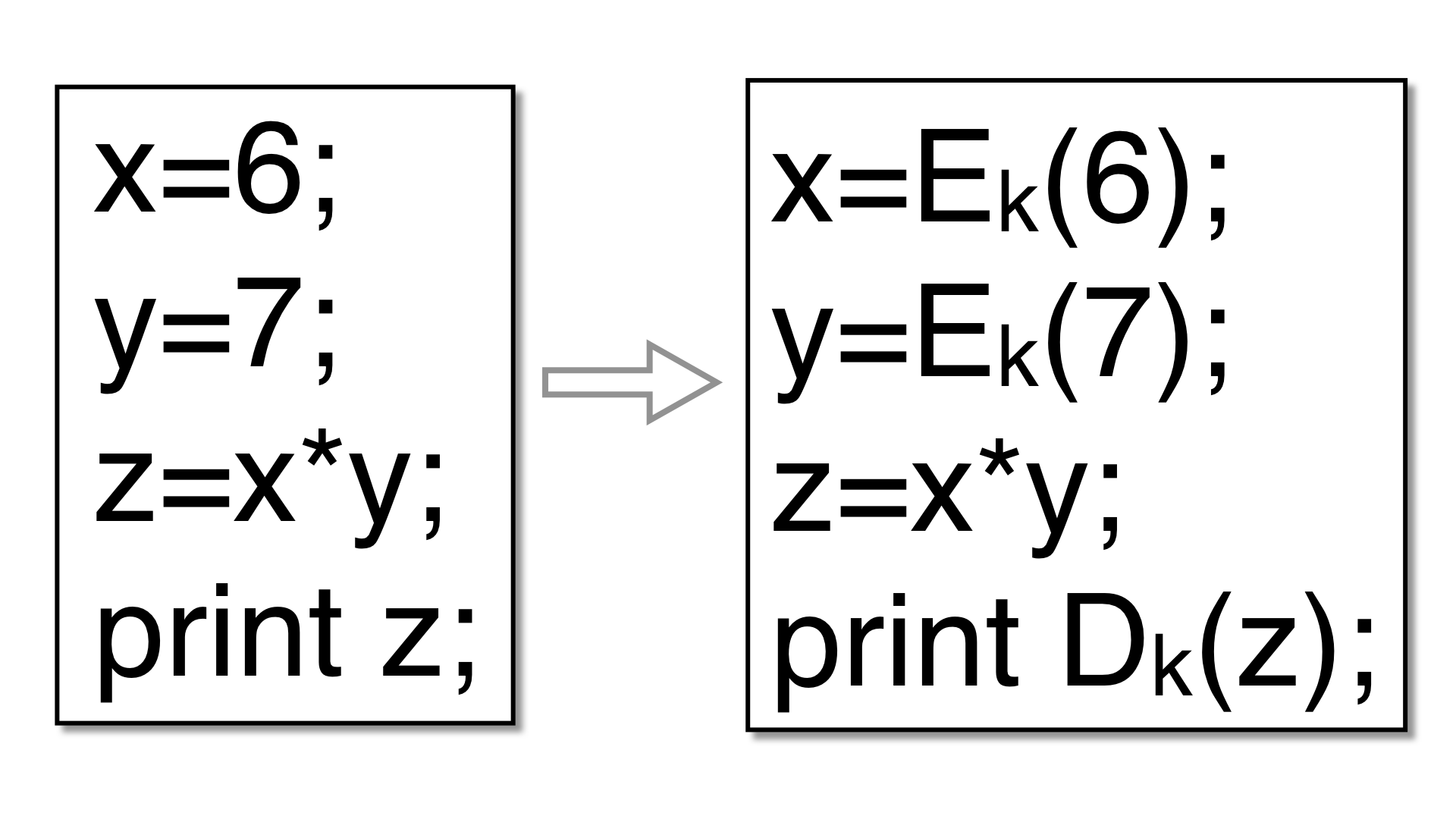# Encode Datafree web templates

Encode integer variables so that they have a non-standard data representation. The goal is for a variable's real value (and the values of intermediate expressions used to compute it) to never be revealed, until it is printed or otherwise escapes the program. For example, an integer variable `v` could be replaced with:

``````v' = a*v + b
``````
free web templates

where `a` is a random odd integer and `b` a random integer. For example, given this program

free web templates

``````int main () {
int arg1 = ...
int arg2 = ...
int a = arg1;
int b = arg2;
int x = a*b;
printf("x=%i\n",x);
}
``````
free web templates

Tigress might produce the following:

``````a = 1789355803 * arg1 + 1391591831;
b = 1789355803 * arg2 + 1391591831;
x = ((3537017619 * (a * b) - 3670706997 * a) - 3670706997 * b) + 3171898074;
printf("x=%i\n", -757949677 * x - 3670706997);
``````

### Usage

free web templates

A typical invokation of this transformation lists a collection of local variables and formal parameters, and global variables:

``````   --Transform=EncodeData \
--GlobalVariables='g1,g2' \
--LocalVariables='fun1:L1,L2;fun2:L3' \
--EncodeDataCodecs=poly1
``````
free web templates

These variables should all be integers, pointers to integers, arrays of integers, or combinations of these. In the example above, `g1` may be an `int`, `L1` an `int*`, `L2` an array of `int`s, and `L3` an array of pointers to `int`s.

OptionArgumentsDescription
--Transform EncodeData Replace integer variables with a different encoding. Use --GlobalVariables and --LocalVariables to specify the variables that should be transformed. In addition to the variables specifed, any other variables that are related through aliasing will be transformed. Only integer variables, arrays of integers, and pointers to integers are currently supported. Avoid structs, since our alias analysis algorithm conflates all fields.
--EncodeDataCodecs poly1, xor, add, * Comma-separated list of the kinds of codecs that may be used. Only poly1 currently makes sense; avoid the others. Default=poly1.
• poly1 = Linear transformation of the form a*x+b.
• xor = Exclusive-or with a constant.
• add = Add a constant and promote to next largest integer type. Will fail for the largest integer type.
• * = Same as poly1,xor,add

### Issues

free web templates

If you ask Tigress to encode a variable `x`, then all variables related to `x` through aliasing must also be encoded. In cases where our alias analysis algorithm isn't precise enough, encoding will fail at transformation time. For large programs, the alias analysis routine may take a very long time.

### References

free web templates

This transformation is based on ideas from several Cloakware/IRDETO papers and patents: